Draft-algebra II unit 1-Polynomial, Rational and Radical Relationships

 Page 1/10 Date conversion 02.05.2018 Size 0.49 Mb.

DRAFT-Algebra II Unit 1–Polynomial, Rational and Radical Relationships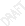Algebra II Unit 1 Snap Shot Unit Title Content Overview Standards in this Unit Unit 1 Polynomial, Rational and Radical Relationships Perform arithmetic operations with complex numbers. Use complex numbers in polynomial identities and equations. Interpret the structure of expressions. Write expressions in equivalent forms to solve problems. Perform arithmetic operations on polynomials. Understand the relationship between zeros and factors of polynomials. Use polynomial identities to solve problems. Rewrite rational expressions. Understand solving radical, rational and polynomial equations as a process of reasoning and explain the reasoning. Represent and solve equations and inequalities graphically including systems which are comprised of a variety of functions. Analyze polynomial and rational functions using different representations. N.CN.1 N.CN.2 N.CN.7 N.CN.8 (+) N.CN.9 (+) A.SSE.1 A.SSE.2 A.SSE.3* A.SSE.4 A.APR.1 A.APR.2 A.APR.3 A.APR.4 A.APR.5(+) A.APR.6 A.APR.7(+) A.REI.2 A.REI.11 F.IF.7 *Indicates standards added to Algebra II Unit 1 by Maryland

Overview

The overview is intended to provide a summary of major themes in this unit.
Algebra II Unit 1develops the structural similarities between the system of polynomials and integers within the system of real numbers. Students draw on analogies between polynomial arithmetic and base-ten computation, focusing on properties of operations, particularly the distributive property. Students connect multiplication of polynomials with multiplication of multi-digit integers, and division of polynomials with long division of integers. Students identify zeros of polynomials, including complex zeros of quadratic polynomials, and make connections between zeros of polynomials and solutions of polynomial equations. The unit culminates with the fundamental theorem of algebra. Rational numbers extend the arithmetic of integers by allowing division by all numbers except 0. Similarly, rational expressions extend the arithmetic of polynomials by allowing division by all polynomials except the zero polynomial. A central theme of this unit is that the arithmetic of rational expressions is governed by the same rules as the arithmetic of rational numbers.

Teacher Notes

The information in this component provides additional insights which will help the educator in the planning process for the unit.

• Polynomial, rational and radical relationships must be explored algebraically, numerically and graphically to help students attain full conceptual development.

• Coefficients of the polynomials used when performing arithmetic operations on polynomials should not be limited to integers but be expanded to the set of real numbers.

• The cluster statement that reads “Represent and solve equations and inequalities graphically including systems which are comprised of a variety of functions” will be revisited many times. Inequalities are not addressed in this unit.

Note: Algebra I, Geometry, and Algebra II units were created using the model from Appendix A of the Common Core State Standards for these courses. Nationally, a discussion is currently taking place which may result in moving some standards to different courses. Once the national discussion has been finalized, the corresponding assessment will be finalized. At that time, we will edit the units as needed.

Enduring Understandings

Enduring understandings go beyond discrete facts or skills. They focus on larger concepts, principles, or processes. They are transferable and apply to new situations within or beyond the subject . Bolded statements represent Enduring Understandings that span many units and courses. The statements shown in italics represent how the Enduring Understandings might apply to the content in Unit 1 of Algebra II.

• Rules of arithmetic and algebra can be used together with notions of equivalence to transform equations and inequalities.

• For a given set of numbers there are relationships that are always true and these are the rules that govern arithmetic and algebra

• Similarities exist between base-ten computation and the arithmetic of polynomials

• Similarities exist between the arithmetic of rational numbers and the arithmetic of rational expressions.

• Relationships between quantities can be represented symbolically, numerically, graphically and verbally in the exploration of real world situations

• The coordinates of the point(s) where the graphs of equations intersect represent the solution(s) to the system of equations formed by the equations.

• There is a connection between the zeros of the polynomial and solutions of polynomial equations.

• Relationships can be described and generalizations made for mathematical situations that have numbers or objects that repeat in predictable ways.

• Multiple representations may be used to model given real world relationships.

• Mathematics can be used to solve real world problems and can be used to communicate solutions to stakeholders.

Essential Question(s)

A question is essential when it stimulates multi-layered inquiry, provokes deep thought and lively discussion, requires students to consider alternatives and justify their reasoning, encourages re-thinking of big ideas, makes meaningful connections with prior learning, and provides students with opportunities to apply problem-solving skills to authentic situations. Bolded statements represent Essential Questions that span many units and courses. The statements shown in italics represent Essential Questions that are applicable specifically to the content in Unit 1 of

Algebra II.

• When and how is mathematics used in solving real world problems?

• What characteristics of problems would determine how to model the situation and develop a problem solving strategy?

• What is the role of complex numbers in the equation solving process?

• When and why is it necessary to follow set rules/procedures/properties when manipulating numeric or algebraic expressions?

• How do the ordered pairs on the graph of an equation relate to the equation itself and then to a system which contains the given equation?

• What are some similarities and differences between the algorithms used for performing operations on rational numbers and the algorithms used for performing operations on rational expressions?

• Why does the equation solving process sometimes produce extraneous solutions?

Possible Student Outcomes

The following list provides outcomes that describe the knowledge and skills that students should understand and be able to do when the unit is completed. The outcomes are often components of more broadly-worded standards and sometimes address knowledge and skills related to the standards. The lists of outcomes are not exhaustive, and the outcomes should not supplant the standards themselves. Rather, they are designed to help teachers “drill down” from the standards and augment as necessary, providing added focus and clarity for lesson planning purposes. This list is not intended to imply any particular scope or sequence.
N.CN.1 Know there is a complex number i such that i2 = −1, and every complex number has the form a + bi with a and b real.

The student will:

• know that the definition of the imaginary unit is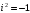and is used to express the square root of a negative number

• write complex numbers in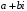form and identify the real and imaginary parts of the number.

N.CN.2 Use the relation i2 = –1 and the commutative, associative, and distributive properties to add, subtract, and multiply complex numbers.

The student will:

• perform operations on complex numbers.

• simplify algebraic expressions that contain complex numbers.

N.CN.7 Solve quadratic equations with real coefficients that have complex solutions.

Note: Limit to polynomials with real coefficients.
The student will:

• solve quadratic equations that have complex solutions.

• describe the nature of the roots of a quadratic equation by analyzing the discriminant or looking at the graph of the quadratic function.

N.CN.8 (+) Extend polynomial identities to the complex numbers. For example, rewrite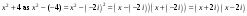The student will:

• (+)factor polynomials using complex numbers

• (+)extend polynomial identities to complex numbers

N.CN.9 (+) Know the Fundamental Theorem of Algebra; show that it is true for quadratic polynomials.

Cluster Note: Extend to polynomial and rational expressions.
The student will:

A.SSE.1 Interpret expressions that represent a quantity in terms of its context.

a. Interpret parts of an expression, such as terms, factors, and coefficients.

b. Interpret complicated expressions by viewing one or more of their parts as a single entity.

For example, interpret P(1+r)n as the product of P and a factor not depending on P.
The student will:

• interpret (assign meaning to) coefficients, constants & variables of polynomial & rational expressions.

• use the leading coefficient of a polynomial to discuss the end behavior of the polynomial.

• use factors of a polynomial to identify the x-intercepts of the graph of the polynomial.

A.SSE.2 Use the structure of an expression to identify ways to rewrite it. For example, see x4 – y4 as (x2)2 – (y2)2, thus recognizing it as a difference of squares that can be factored as (x2 – y2)(x2 + y2).

The student will:

• simplify polynomial expressions.

• factor polynomials based upon the structure of the expression.

A.SSE.4 Derive the formula for the sum of a finite geometric series (when the common ratio is not 1), and use the formula to solve problems. For example, calculate mortgage payments.

Note: Consider extending this standard to infinite geometric series in curricular implementations of this course description.

The student will:

• identify and define geometric series.

• derive and apply the formula for the sum of a finite geometric series.

A.APR.1 Understand that polynomials form a system analogous to the integers, namely, they are closed under the operations of addition, subtraction, and multiplication; add, subtract, and multiply polynomials.

The student will:

• add, subtract, and multiply polynomials.

• describe how closure applies to polynomials of degree > 2.

A.APR.2 Know and apply the Remainder Theorem: For a polynomial p(x) and a number a, the remainder on division by x – a is p(a), so p(a) = 0 if and only if (x – a) is a factor of p(x).

The student will:

• identify and apply the Remainder Theorem.

• solve polynomial equations.

• solve polynomial equations using synthetic division

• factor polynomials using the Remainder Theorem

A.APR.3 Identify zeros of polynomials when suitable factorizations are available, and use the zeros to construct a rough graph of the function defined by the polynomial.

Cluster Note: This cluster has many possibilities for optional enrichment, such as relating the example in A.APR.4 to the solution of the system u2+v2=1, v = t(u+1), relating the Pascal triangle property of binomial coefficients to (x+y)n+1 = (x+y)(x+y)n, deriving explicit formulas for the coefficients, or proving the binomial theorem by induction.
The student will:

• use the factors of a given polynomial to identify it’s zeros

• make a rough sketch of the graph of a polynomial using zeros and end behavior

A.APR.4 Prove polynomial identities and use them to describe numerical relationships.

For example, the polynomial identity (x2 + y2)2 = (x2 – y2)2 + (2xy)2 can be used to generate Pythagorean triples.
The student will:

• prove polynomial identities.

• use polynomial identities to describe numerical relationships.

A.APR.5 (+) Know and apply the Binomial Theorem for the expansion of (x + y)n in powers of x and y for a positive integer n, where x and y are any numbers, with coefficients determined for example by Pascal’s Triangle.

Note: The Binomial Theorem can be proved by mathematical induction or by combinatorial argument.
The student will:

• (+) recognize and apply the Binomial Theorem.

A.APR.6 Rewrite simple rational expressions in different forms; write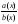in the form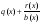where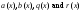are polynomials with the degree of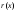less than the degree of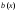, using inspection, long division, or, for the more complicated examples, a computer algebra system.

Note: The limitations on rational functions apply to the rational expressions in this standard. Limitations: In this course rational functions are limited to those whose numerators are of degree at most one and denominators of degree at most 2.

The student will:

• simplify rational expressions.

• divide polynomials using the long division.